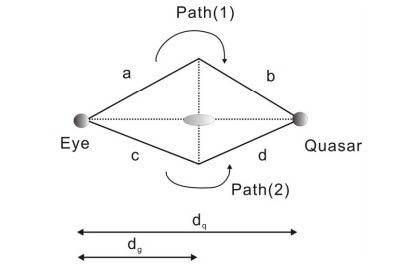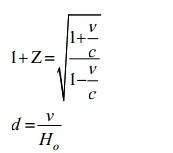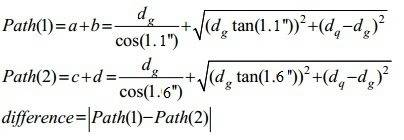# Solving Gravitational Lensing Homework for Hubble Constant

• MrSavikins
Arcseconds are radians. So the answer would be 0.00216516 * (1.6378 * 10^-5) = 6.719x10^-5 light years.In summary, the quasar is lensed and the two images are separated by 1.1 and 1.6 arcseconds. The difference in magnitude does not matter, but the time lag between the two flares does.f

## Homework Statement

A quasar at redshift 0.3 is gravitationally lensed into two images by an elliptical galaxy at redshift 0.18. The two images of the quasar are separated from the center of the galaxy by 1.1 and 1.6 arcseconds, on opposite sides. One of the quasar images flares up in intensity by 1 magnitude and 16.7 days later the second quasar image flares by the same amount and with the same time profile. Making the approximation that the quasar lies directly behind the galaxy, derive a value for the Hubble Constant.
Ignore acceleration and expansion of universe and galaxies. Assume paths are straight lines.

## Homework Equations

Posted as attached files with a beautiful diagram of the situation.

## The Attempt at a Solution

So I started by plugging in v and c in the redshift equation. I then put the value I got for v into the d=v/H equation and kept H as it is because that's what we're trying to derive.
I got v_g and v_q and then d_g and d_q in terms of H_0.
I then plugged all my values into my path equations and received a path difference of 0.00216516/H_0 (you guys can test me on this I spent a long time trying to get to this value so it could be wrong).

I don't know what to do from here because I have no idea what a difference in magnitude and a time given to us has to do with the path difference. If I could relate the time lag between the two flares with the path difference then I can get my H_0 value yay.If I could relate the time lag between the two flares with the path difference then I can get my H_0 value yay.
Light moves at the speed of light...

The difference in magnitude does not matter. To verify you actually see the same quasar twice, it is nice to check that both images follow the same profile, but you don't have to care about that here.

and received a path difference of 0.00216516/H_0
This is a time, not a distance.

Thanks! Yeah I did that, and I set that value equal to the light days difference. Also it turns out I made a mistake and put in arcseconds into the cosine and tangent functions and I actually should have converted the arcseconds to radians first and then put them into the trig functions.